Something interesting, old thread - page 8704

Doc

This would be a simplest way to calculate MACD. But, it is hard to limit it to just certain number of bars (EMA needs some bars to claculate before "stabilizing") Anyway, try it out. It is even a correct MACD (again built in MACD is using SMA instead of EMA for signal line, and original MACD should use EMA for signal line)138821

Limiting macd calculation

Doc

This is one possible solution. Macd is made as a new function (it does not use the built in function) And can be limited in calculation. Here is a comparison of the previous "simple" macd and this one with limited bars to calculate. When you decide how many bars you want to calculate, give it some room (the example is using 200 bars limit, and you can see that at the beginning they are different, and that towards the end, eventually they are the same. It is due to the cumulative effect of ema calculation used for macd (ema always uses previous value so it depends on where does it start)).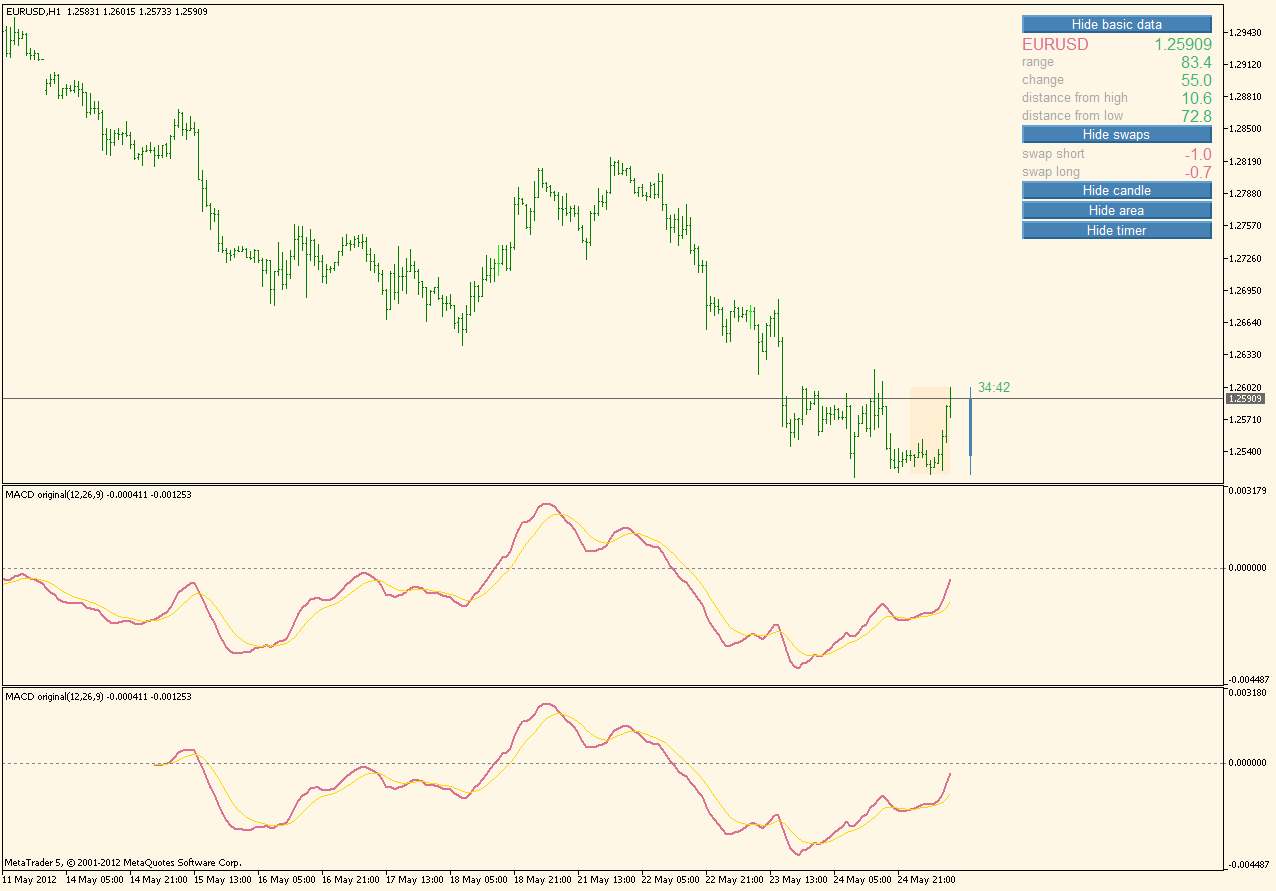Files:704

Doc

This is one possible solution. Macd is made as a new function (it does not use the built in function) And can be limited in calculation. Here is a comparison of the previous "simple" macd and this one with limited bars to calculate. When you decide how many bars you want to calculate, give it some room (the example is using 200 bars limit, and you can see that at the beginning they are different, and that towards the end, eventually they are the same. It is due to the cumulative effect of ema calculation used for macd (ema always uses previous value so it depends on where does it start)).

I love it!

but a question is needed...

This is the iMacd function: iMACD(name,period,fast_ema_period,slow_ema_period,signal_period,applied_price);

So where is the metaquotes explanation for your ...tmacd,tsign,i,rates_total,MathMin(rates_total,InpBarsToCalc),0);Can you recommend a good ebook?138821

...It is different than the built in one (it is not even made to be the same as the built in one). It also calculates signal as EMA (as Gerald Appel did it in his original and as almost all normal trading platforms do)

First of all it can calculate MACD of any value (macd of rsi, cci, any other indi, price, anything), not just the prices (first parameter is called "price" but you can pass it any value and it will calculate the MACD of it). So the parameters would go like this :

- "price" - the value to use in calculating the MACD

- "fastEMA" - fast ema period

- "slowEMA" - slow ema period

- "signalEMA" - signal ema period

- "macd' - a variable that will receive macd value

- "signal" - a variable that will receive signal of macd value

- "i" - index (bar) to calculate (0== oldest, rates_tola==newest (current) bar)

- "totalBars" total bars on chart

- "barsToCalculate" - number of bars that you want actually to calculate MACD for

- "instanceNo" - instance number

So this is "function" returns 2 values instead of just one with a single call : "macd" and "signal" are passed by reference and they are assigned values. "totalBars" needs to be passed in order to make the function know exactly how many bars are there on the chart (it could be done differently, but this is the fastest way). The bars to calculate is I think obvious. The "instanceNo" is the one that needs to be explained

Imagine you want 2 MACDs calculate. Simply set the "totalIBuffers" in code to 6 and than calculate something like "iMacd(value1,...,0) and iMacd(value2,...,1) and you will have 2 separate macd calculations that do not intermix. The only "bad" thing of this approach is that you have to know in advance how many different macd values are you going to calculate, but other than that, there is no other "bad" things. It is still just one instance of the function that is calculati9ng all the values and all it does is allocate its internal calculating buffers (you even do not have to know about them, except to adjust the number of buffers used) and all the rest will be done by the function

dr.house7:
I love it!

but a question is needed...

This is the iMacd function: iMACD(name,period,fast_ema_period,slow_ema_period,signal_period,applied_price);

So where is the metaquotes explanation for your ...tmacd,tsign,i,rates_total,MathMin(rates_total,InpBarsToCalc),0);Can you recommend a good ebook?704

:)

It is different than the built in one (it is not even made to be the same as the built in one). It also calculates signal as EMA (as Gerald Appel did it in his original and as almost all normal trading platforms do)...

...

This is a great explanation, and this is an amazing indicator!I really don't understand how metaeditor allocate buffers but anyway I need to study much more.

You said "it can calculate MACD of any value (macd of rsi,etc..." could I see a macd of rsi? Just to better understand138821

Doc

This would be a macd of a rsi. You will find that is quite easy to use that macd for those type of calculations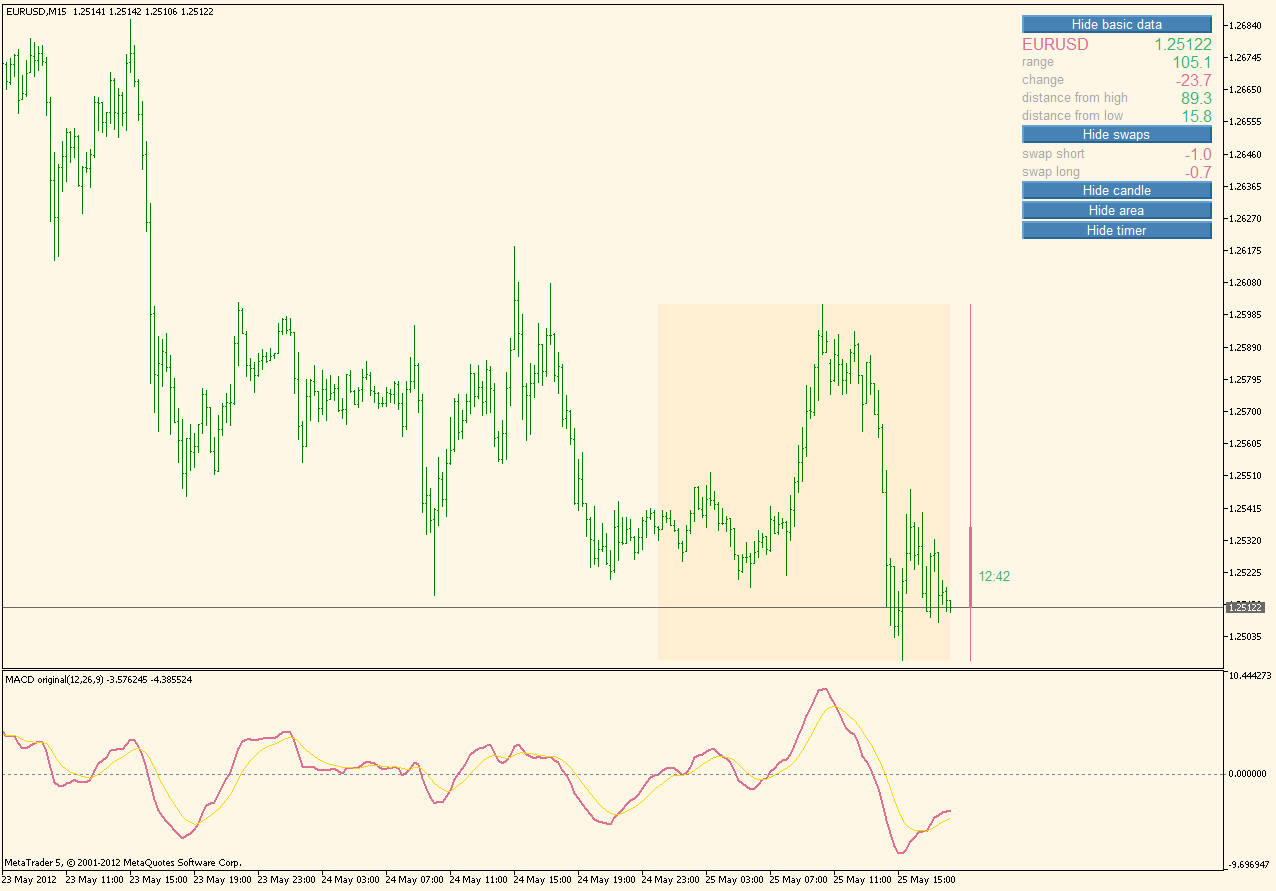PS: the whole "trick" is that I do not use what metatrader calls buffers at all for these calculations. All the work is done in arrays, and that way we have no limit whatsoever (as the number of buffers is limited even in metatrader 5 version)

dr.house7:
This is a great explanation, and this is an amazing indicator!I really don't understand how metaeditor allocate buffers but anyway I need to study much more. You said "it can calculate MACD of any value (macd of rsi,etc..." could I see a macd of rsi? Just to better understand
Files:704

Doc

This would be a macd of a rsi. You will find that is quite easy to use that macd for those type of calculations

PS: the whole "trick" is that I do not use what metatrader calls buffers at all for these calculations. All the work is done in arrays, and that way we have no limit whatsoever (as the number of buffers is limited even in metatrader 5 version)

Tooooo difficultSo this is an ema rsi with ema signal? I thought the macd of rsi was a normal rsi with an ema signal. About buffers...yes I saw you used only 2 buffers 1 for macd and the other for signal, but I don't understand how metaeditor in other indicators arrange all the buffers...I'm very disappointed that is not possible to change directly from metaeditor the source code of main indicators such as cci rsi etc. The only way is to crack the platform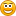By the way, did you see the disaster code of common indicators in mql5 example folder? How many buffers they use? With multiple cci into the chart, my cpu usage goes very very high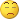138821

...

Doc

Nagh. It is a macd of rsi with an ema of a macd of rsi (the signal line)

As of code : unfortunately their examples and their code is not known for clarity, simplicity nor efficiency. Somehow that (the clarity, simplicity and efficiency) was never a priority for them

dr.house7:
Tooooo difficultSo this is an ema rsi with ema signal? I thought the macd of rsi was a normal rsi with an ema signal. About buffers...yes I saw you used only 2 buffers 1 for macd and the other for signal, but I don't understand how metaeditor in other indicators arrange all the buffers...I'm very disappointed that is not possible to change directly from metaeditor the source code of main indicators such as cci rsi etc. The only way is to crack the platformBy the way, did you see the disaster code of common indicators in mql5 example folder? How many buffers they use? With multiple cci into the chart, my cpu usage goes very very high138821

MACD of CCI ...

As a continuation of a "MACD of ..." series, here is a MACD of CCI. It is using the CCI simple way of calculation and the MACD function described in one of the previous posts to do that (so it does not use anything "outside" this indicator, the whole code is within it) Compared to "regular" MACD with default parameters it is much "faster", so one might consider using longer CCI period calculation in order to slow it down a bit. Here is an example of a MACD of a 32 period CCI (upper) compared to regular MACD(lower).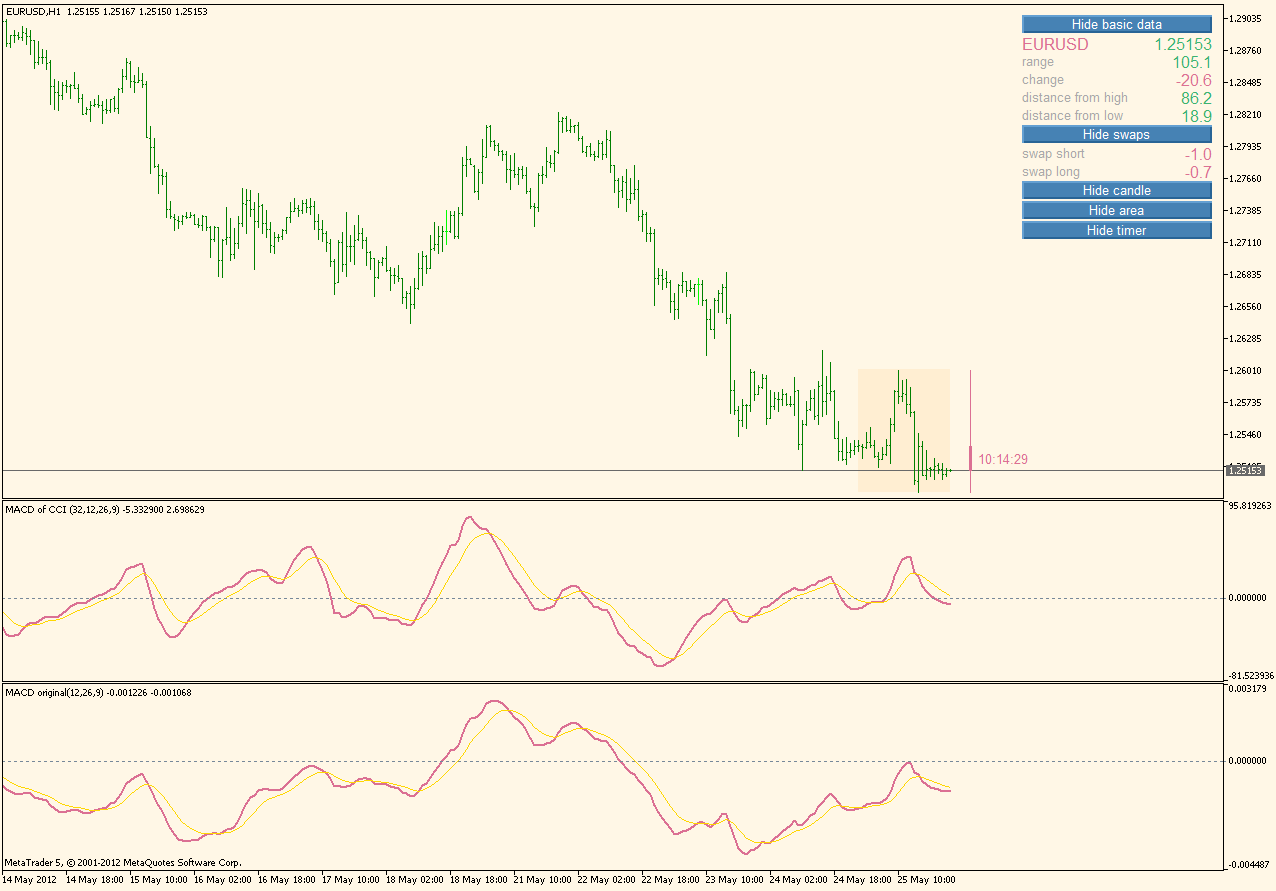Even like that MACD of CCI is faster than the regular MACD, so one needs to experiment a bit with parameters to adjust it to trading style needs (for scalping, probably best to use shorter CCI calculating periods, for trend trading probably best to use longer CCI calculating periods)

Files:704

black holes

...just for talk...

Did you see the cross eur/usd? How is it possible something like this (57 pips!)? Where is the lost data?

I mean, in the stock market you can follow the data also when the market is closed (and when all derivates still open), so the movement is continuos (for example try it on the platform "think or swim") without similar black holes.

So why the forex market is still ..."dark"?doc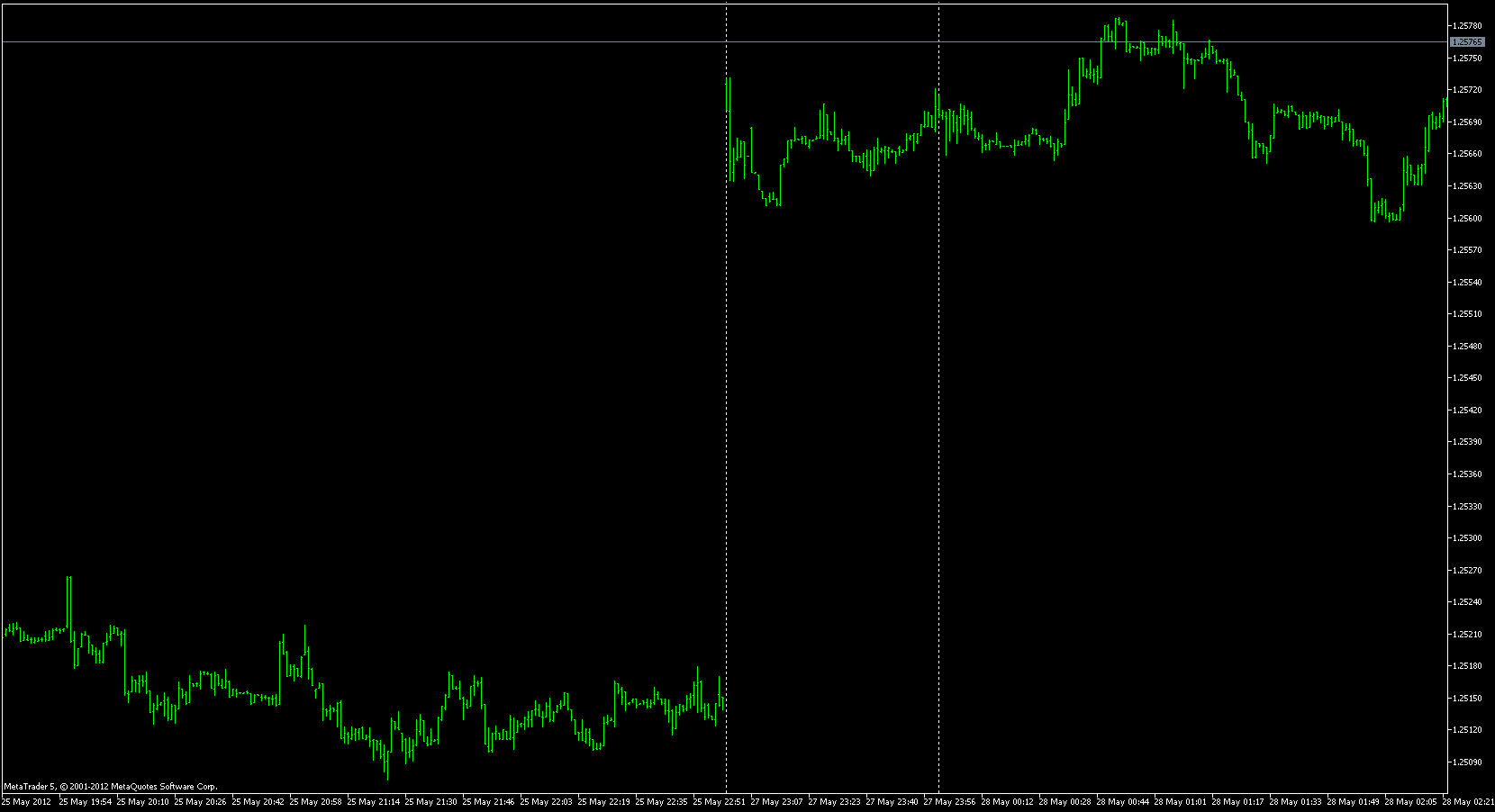Files:
eurusdm1.png 61 kb